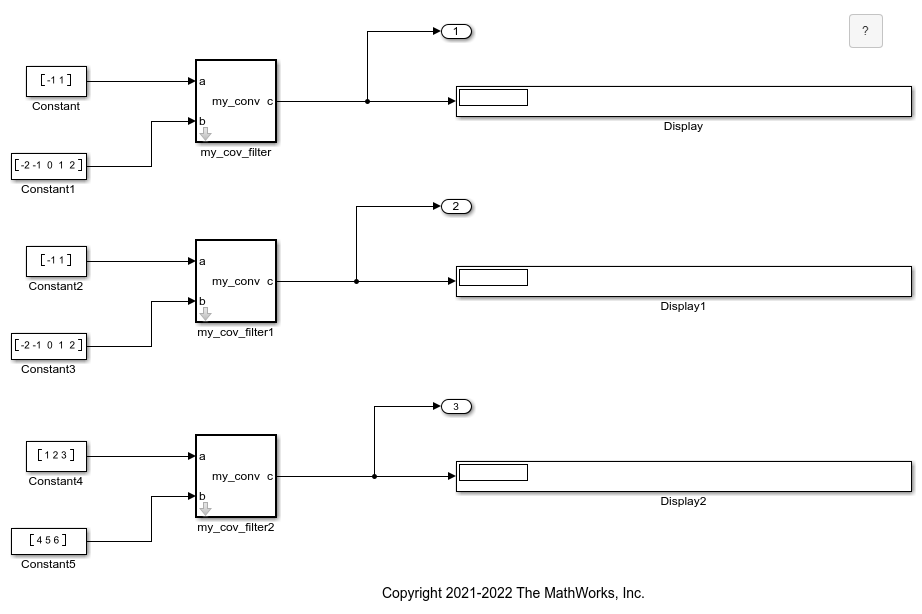# Run Simulations and Generate Code with Masked Library Subsystems Containing MATLAB Function Blocks

This example shows how to use a masked library subsystem that contains a MATLAB Function block to run simulations and generate C code. Edits to the function code, the subsystem, or the mask properties affect all instances of the block in the model, while changes to the mask parameter affect only the selected block. Use masked library blocks if you need duplications of custom blocks that can generate code. For more information, see Create Custom Library and Design and Create a Custom Block.### Navigate the Model

The masked subsystem contains a MATLAB Function block that uses the `conv` function to calculate the convolution of two input vectors. The mask parameter shape allows you to change the normalization weight to `full`, `same`, or `valid`. The block calculates the convolution using this MATLAB® code:

```function c = my_conv(a, b, shape) if shape == 1 c = conv(a, b, "full"); elseif shape == 2 c = conv(a, b, "same"); else c = conv(a, b, "valid"); end ```

In this model, the shape parameter of the top instance of the block is set to `full`, the middle instance is set to `same`, and the bottom instance is set to `valid`. Run the simulation to calculate the convolution of the vectors and display their values in the Display blocks.

The two top block instances output different vector sizes because each block calculates the convolution with a different weight. Change the shape parameter in any of the blocks and simulate again to see the output change.

### Generate C Code From the Model

If you have a license for Embedded Coder® or Simulink® Coder™, you can generate code from this example without changing the parameters or the blocks. However, MATLAB Function blocks support code generation for only a restricted subset of MATLAB functions. For more information, see Functions and Objects Supported for C/C++ Code Generation (MATLAB Coder).

If the parameters and inputs are identical for multiple instances of the library blocks, you can generate code with reusable functions that represent each identical MATLAB Function block. You can enable this behavior by right-clicking the MATLAB Function block, clicking Block Parameters (Subsystem), and then opening the Code Generation tab. Set Function packaging to `Reusable function`. For more information, see Generate Reusable Code from Library Subsystems Shared Across Models (Simulink Coder).

Generate code by opening the Embedded Coder or Simulink Coder apps and clicking Generate Code. This model generates unique C functions for each block instance. To produce reusable functions, set the shape parameter to `full` for each library subsystem instance and generate code. The generated code reuses logic for the `my_conv_filter` and `my_conv_filter1` instances because they have the same block inputs and mask parameter value.

``` /* * Output and update for atomic system: * '<S1>/MATLAB Function' * '<S2>/MATLAB Function' */ void MLFB_model_MATLABFunction(const real_T rtu_a, const real_T rtu_b, real_T rty_c) { int32_T b_k; int32_T i; for (i = 0; i < 6; i++) { rty_c[i] = 0.0; }```
``` for (i = 0; i < 2; i++) { for (b_k = 0; b_k < 5; b_k++) { int32_T tmp; tmp = i + b_k; rty_c[tmp] += rtu_a[i] * rtu_b[b_k]; } } }```

The `my_conv_filter2` instance uses a different function because it uses different inputs.

``` /* Output and update for atomic system: '<S3>/MATLAB Function' */ void MLFB_model_MATLABFunction_p(const real_T rtu_a, const real_T rtu_b, real_T rty_c) { int32_T i; for (i = 0; i < 5; i++) { rty_c[i] = 0.0; }```
``` for (i = 0; i < 3; i++) { rty_c[i] += rtu_b[i] * rtu_a; rty_c[i + 1] += rtu_b[i] * rtu_a; rty_c[i + 2] += rtu_b[i] * rtu_a; } }```